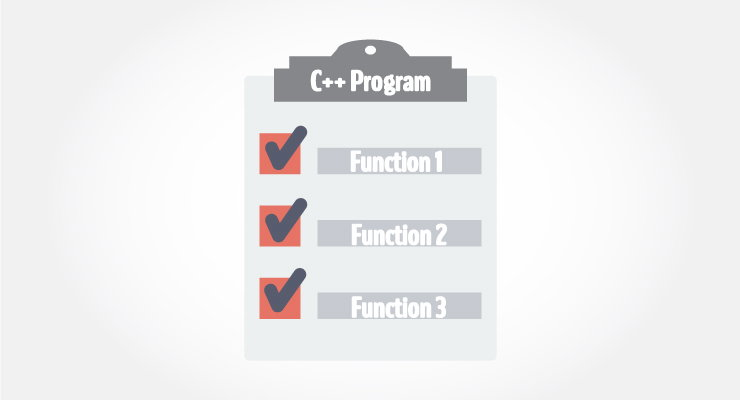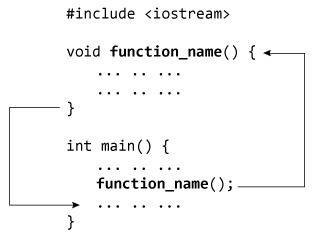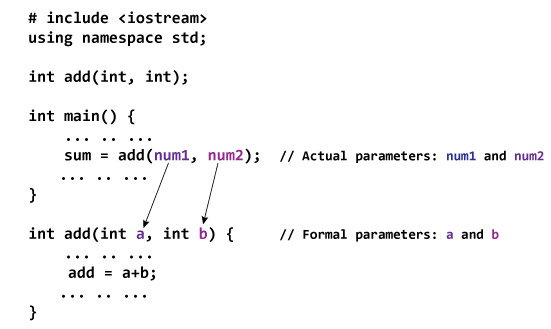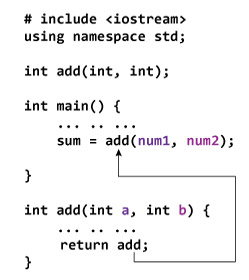# C++ Functions

In this article, you'll learn everything about functions in C++; what type of functions are there, how to use them with examples.In programming, function refers to a segment that groups code to perform a specific task.

Depending on whether a function is predefined or created by programmer; there are two types of function:

1. Library Function
2. User-defined Function

## Library Function

Library functions are the built-in function in C++ programming.

Programmer can use library function by invoking function directly; they don't need to write it themselves.

### Example 1: Library Function

``````#include <iostream>
#include <cmath>

using namespace std;

int main()
{
double number, squareRoot;
cout << "Enter a number: ";
cin >> number;

// sqrt() is a library function to calculate square root
squareRoot = sqrt(number);
cout << "Square root of " << number << " = " << squareRoot;
return 0;
}``````

Output

```Enter a number: 26
Square root of 26 = 5.09902```

In the example above, `sqrt()` library function is invoked to calculate the square root of a number.

Notice code `#include <cmath>` in the above program. Here, `cmath` is a header file. The function definition of `sqrt()`(body of that function) is present in the cmath header file.

You can use all functions defined in `cmath` when you include the content of file `cmath` in this program using `#include <cmath>` .

Every valid C++ program has at least one function, that is, `main()` function.

## User-defined Function

C++ allows programmer to define their own function.

A user-defined function groups code to perform a specific task and that group of code is given a name(identifier).

When the function is invoked from any part of program, it all executes the codes defined in the body of function.

### How user-defined function works in C Programming?Consider the figure above.

When a program begins running, the system calls the `main()` function, that is, the system starts executing codes from `main()` function.

When control of the program reaches to `function_name()` inside `main()`, it moves to `void function_name() `and all codes inside `void function_name()` is executed.

Then, control of the program moves back to the main function where the code after the call to the `function_name()` is executed as shown in figure above.

### Example 2: User Defined Function

C++ program to add two integers. Make a function `add()` to add integers and display sum in main() function.

``````#include <iostream>
using namespace std;

// Function prototype (declaration)
int add(int, int);

int main()
{
int num1, num2, sum;
cout<<"Enters two numbers to add: ";
cin >> num1 >> num2;

// Function call
sum = add(num1, num2);
cout << "Sum = " << sum;
return 0;
}

// Function definition
int add(int a, int b)
{
int add;
add = a + b;

// Return statement
return add;
}``````

Output

```Enters two integers: 8
-4
Sum = 4```

### Function prototype (declaration)

If a user-defined function is defined after `main()` function, compiler will show error. It is because compiler is unaware of user-defined function, types of argument passed to function and return type.

In C++, function prototype is a declaration of function without its body to give compiler information about user-defined function. Function prototype in the above example is:

`int add(int, int);`

You can see that, there is no body of function in prototype. Also, there are only return type of arguments but no arguments. You can also declare function prototype as below but it's not necessary to write arguments.

`int add(int a, int b);`

Note: It is not necessary to define prototype if user-defined function exists before `main()` function.

### Function Call

To execute the codes of function body, the user-defined function needs to be invoked(called).

In the above program, `add(num1,num2);` inside `main()` function calls the user-defined function.

The function returns an integer which is stored in variable add.

### Function Definition

The function itself is referred as function definition. Function definition in the above program is:

```// Function definition
int add(int a,int b)
{
int add;
add = a + b;
return add;
}```

When the function is called, control is transferred to the first statement of the function body.

Then, other statements in function body are executed sequentially.

When all codes inside function definition is executed, control of program moves to the calling program.

### Passing Arguments to Function

In programming, argument (parameter) refers to the data which is passed to a function (function definition) while calling it.

In the above example, two variables, num1 and num2 are passed to function during function call. These arguments are known as actual arguments.

The value of num1 and num2 are initialized to variables a and b respectively. These arguments a and b are called formal arguments.

This is demonstrated in figure below:Notes on passing arguments

• The numbers of actual arguments and formals argument should be the same. (Exception: Function Overloading)
• The type of first actual argument should match the type of first formal argument. Similarly, type of second actual argument should match the type of second formal argument and so on.
• You may call function a without passing any argument. The number(s) of argument passed to a function depends on how programmer want to solve the problem.
• You may assign default values to the argument. These arguments are known as default arguments.
• In the above program, both arguments are of `int` type. But it's not necessary to have both arguments of same type.

### Return Statement

A function can return a single value to the calling program using return statement.

In the above program, the value of add is returned from user-defined function to the calling program using statement below:

`return add;`

The figure below demonstrates the working of return statement.In the above program, the value of add inside user-defined function is returned to the calling function. The value is then stored to a variable sum.

Notice that the variable returned, i.e., add is of type `int` and sum is also of int type.

Also, notice that the return type of a function is defined in function declarator `int add(int a, int b)`The `int` before `add(int a, int b)` means the function should return a value of type `int`.

If no value is returned to the calling function then, `void` should be used.